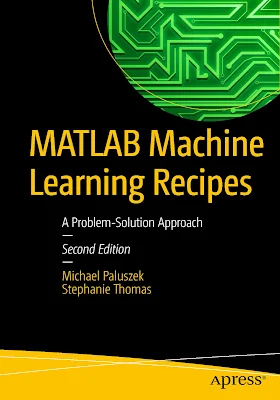# MATLAB Machine Learning Recipes: A Problem-Solution Approach

## MATLAB Machine Learning Recipes: A Problem-Solution Approach

Michael Paluszek

Preference :

Machine learning is a field in computer science where data are used to predict, or respond to, future data. It is closely related to the fields of pattern recognition, computational statistics, and artificial intelligence. The data may be historical or updated in real-time. Machine learning is important in areas such as facial recognition, spam filtering, and other areas where it is not feasible, or even possible, to write algorithms to perform a task. For example, early attempts at filtering junk emails had the user write rules to determine what was junk or spam. Your success depended on your ability to correctly identify the attributes of the message that would categorize an email as junk, such as a sender address or words in the subject, and the time you were willing to spend on tweaking your rules.MATLAB Machine Learning Recipes: A Problem-Solution Approach

Content :
• An Overview of Machine Learning
• Representation of Data for Machine Learning in MATLAB
• MATLAB Graphics
• Kalman Filters
• Fuzzy Logic
• Data Classification with Decision Trees
• Introduction to Neural Nets
• Classification of Numbers Using Neural Networks
• Pattern Recognition with Deep Learning
• Neural Aircraft Control
• Multiple Hypothesis Testing
• Autonomous Driving with Multiple Hypothesis Testing
• Case-Based Expert Systems
• A Brief History of Autonomous Learning